# Two best ways to convert numbers to words in Excel

In this article I will show you two quick and free ways to convert currency numbers into English words in Excel 2019, 2016, 2013 and other versions.

Microsoft Excel is a great program to calculate this and that. It was initially developed to process large data arrays. However, it also lets creating accounting records like invoices, evaluation or balance sheets quickly and effectively.

In more or less solid payment documents it is necessary to duplicate numeric values with their word form. It is much harder to falsify typed numbers than those written by hand. Some swindler can try to make 8000 out of 3000, while it is almost impossible to secretly replace "three" with "eight".

So what you need is not just convert numbers to words in Excel (e.g. 123.45 to "one hundred and twenty three, forty five"), but spell out dollars and cents (e.g. \$29.95 as "twenty nine dollars and ninety nine cents" ), pounds and pence for GBP, euros and eurocents for EUR, etc.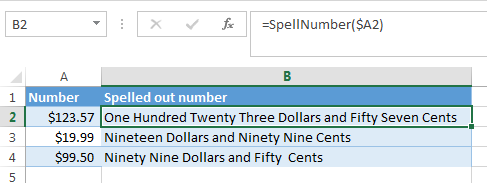Even the latest versions of Excel don't have a built-in tool for spelling numbers, not to mention earlier versions. But that is when Excel is really good. You can always improve its functionality using formulas in all their
combinations, VBA macros, or third-party add-ins.

Below you'll find two ways to convert numbers from figures to words

And, possibly, you may need to convert Words to Numbers in Excel

Note. If you are looking for the number to text conversion, which means you want Excel to see your number as text, it's a bit different thing. For this, you can use the TEXT function or a few other ways described in How to change numbers to text in Excel.

## SpellNumber VBA macro to convert numbers to words

As I have already mentioned, Microsoft didn't want to add a tool for this task. However, when they saw how many users needed it, they created and published the special VBA macro on their website. The macro does what its name SpellNumber suggests. All other macros I came across are based on the Microsoft code.

You can find the macro mentioned as "spellnumber formula". However, it is not a formula, but a macro function, or to be more precise Excel User defined function (UDF).

The spellnumber option is able to write dollars and cents. If you need a different currency, you can change "dollar" and "cent" with the name of your one.

If you are not a VBA savvy guy, below you will find a copy of the code. If you still don't want or haven't time to sort this out, please use this solution.

1. Open the workbook where you need to spell the numbers.
2. Press Alt+F11 to open the Visual Basic editor window.
3. If you have several books opened, check that the needed workbook is active using the list of projects in the upper left corner of the editor (one of the workbook elements is highlighted with blue).
4. In the editor menu go to Insert-> Module.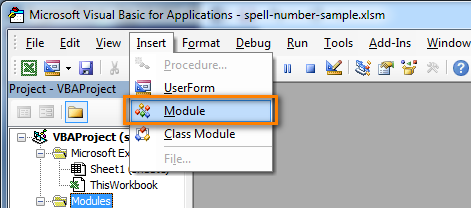5. You should see a window named YourBook - Module1. Select all of the code in the frame below and paste it to this window.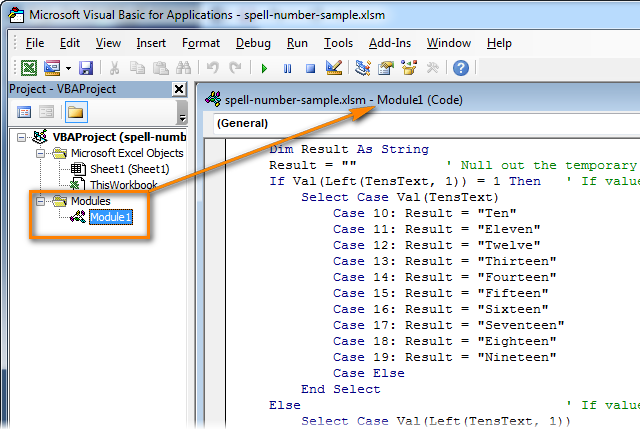6. Press Ctrl+S to save the updated workbook.

You will need to resave your workbook. When you try to save the workbook with a macro you'll get the message "The following features cannot be saved in macro-free workbook"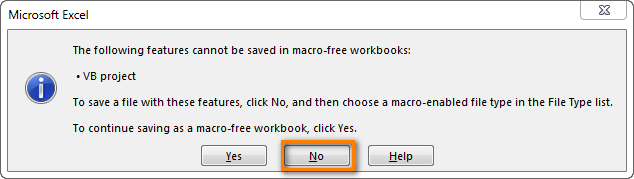Click No. When you see a new dialog, chose the Save as option. In the field "Save as type" pick the option "Excel macro-enabled workbook".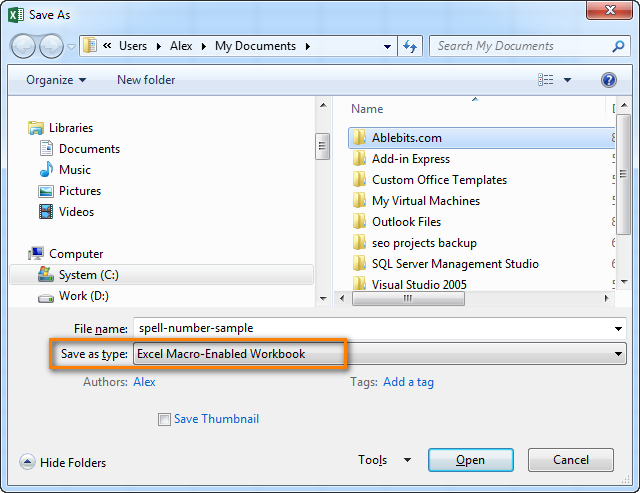#### Use SpellNumber macro in your worksheets

Now you can use the function SpellNumber in your Excel documents. Enter `=SpellNumber(A2)` into the cell where you need to get the number written in words. Here A2 is the address of the cell with the number or amount.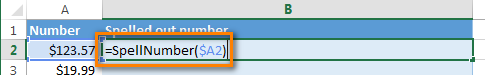Here you can see the result: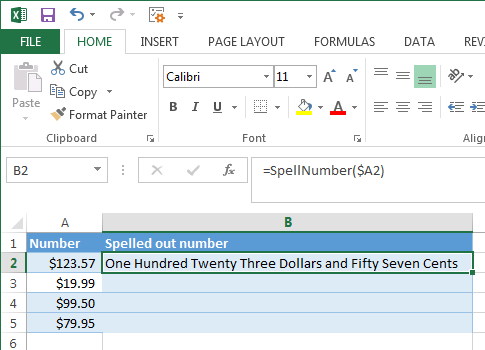Voila!

#### Quickly copy the SpellNumber function to other cells.

If you need to convert the entire table, not just 1 cell, place your mouse cursor to the lower right corner of the cell with the formula until it turns into a small black cross: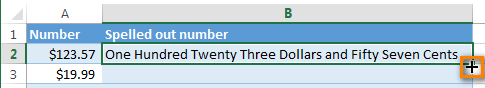Left-click and drag it across the column to fill in the formula. Release the button to see the results: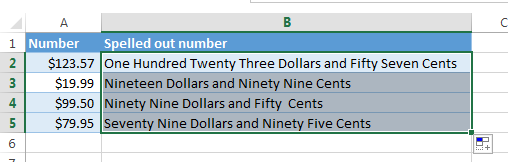Note. Please keep in mind that if you use SpellNumber with a link to another cell, the written sum will be updated each time the number in the source cell is changed.
You can also enter the number directly into the function, for example, `=SpellNumber(29.95)` (29.95 - without quotation marks and the Dollar sign).

#### Disadvantages of using macro to spell numbers in Excel

First off, you must know VBA to modify the code according to your needs. It is necessary to paste the code for each workbook, where you plan to change it. Otherwise, you will need to create a template file with macros and configure Excel to load this file at each start.

The main disadvantage of using a macro is if you send the workbook to somebody else, this person will not see the text unless the macro is built into the workbook. And even if it's built-in, they will get an alert that there are macros in the workbook.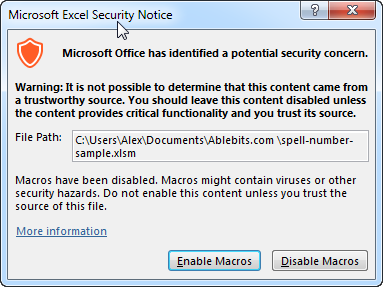## Spell numbers into words using a special add-in

For Excel users who need to quickly spell sums but don't have time to learn VBA or figure out workarounds, we created a special tool that can quickly perform the amount-to-words conversion for a few popular currencies. Please meet the Spell Number add-in included with the latest release of our Ultimate Suite for Excel.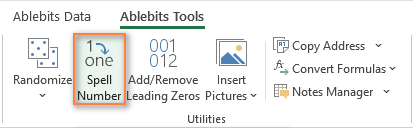Besides being ready for use, the tool is really flexible in converting amounts to text:

• You can select one of the following currencies: USD, EUR, GBP, BIT, AUD.
• Spell the fractional part in cents, pennies, or bitcents.
• Choose any text case for the result: lower case, UPPER CASE, Title Case, or Sentence case.
• Spell the decimal part in different ways.
• Include or omit zero cents.

The add-in supports all modern versions including Excel 365, Excel 2029, Excel 2016, Excel 2013, and Excel 2010. Please feel free to explore other capabilities on the product's home page linked above.

And now, let's see this number spelling utility in action:

1. Select an empty cell for the result.
2. On the Ablebits tab, in the Utilities group, click Spell Number.
3. In the Spill Number dialog window that appears, configure the following things:
• For the Select your number box, pick the cell containing the amount you want to get written as text.
• Specify the desired currently, letter case and the way the decimal part of the number should be spelled.
• Define whether to include zero cents or not.
• Choose whether to insert the result as a value or formula.
4. At the bottom of the dialog window, preview the result. If you are happy with the way your number is written, click Spell. Otherwise, try different settings.

The screenshot below demonstrates the default choices and the spelled number in B2. Please notice a formula (more precisely, a user-defined function) in the formula bar: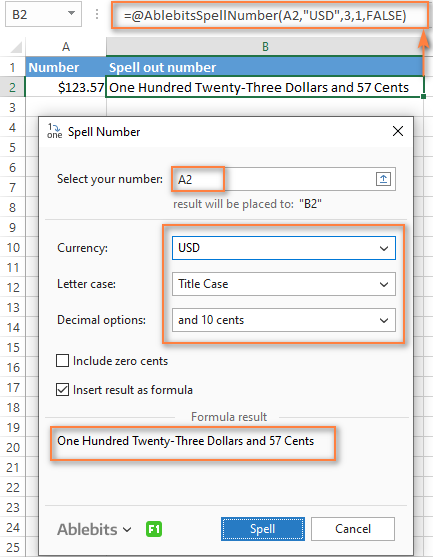And this is a quick demonstration of how other currencies can be spelled out: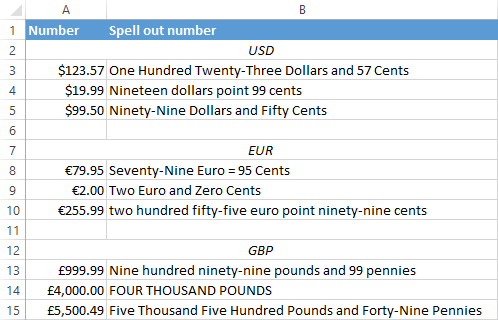Tips and notes:

• Because the Spell Number add-in was designed to handle real-life use cases such as invoices and other financial documents, it can only convert one number at a time.
• To spell a column of numbers, insert a formula in the first cell, and then copy the formula down.
• If there is chance that your source data may change in the future, it's best to insert the result as formula, so it updates automatically as the original number changes.
• When choosing the result as formula option, a custom user-defined function (UDF) is inserted. If you plan to share your workbook with someone who does not have the Ultimate Suite installed, remember to replace formulas with values before sharing.

## Reverse conversion - English words into numbers

Frankly, I can't imagine why you may need it. Just in case… :)

It appears that Excel MVP, Jerry Latham, created such Excel User defined function (UDF) as WordsToDigits. It converts English words back to number.

You can download Jerry's WordsToDigits workbook to see the UDF code. Here you'll also find his examples of how to use the function.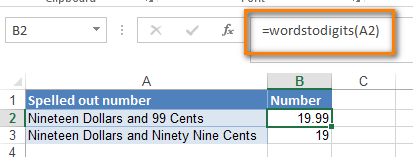You can see how the function works on the sheet "Sample Entries", where you will also be able to enter your own examples. If you plan to employ WordsToDigits in your documents, please be informed that this function has restrictions. For example, it doesn't recognize fractions entered in words. You will find all the details on the "Information" sheet.

## You may also be interested in

1. Thanks from yours formula

2. hi can you help me

I want to convert amount like this;

Php. 1,982.56

one thousand nine hundred eighty two pesos & 56/100 only

3. I'd like to convert the digits to words but without the "dollar or cents"
i.e. 342,880 will be:
"Three Hundred Forty Two Thousand Eight Hundred and Eighty"
and not
"Three Hundred Forty Two Thousand Eight Hundred Eighty Dollars and No Cents"

how do I do that? thank you!

4. Hi, Please insert INR in currency too.

5. There's Error ????

Option Explicit

'Main Function

Function SpellNumber(ByVal MyNumber)

Dim Dollars, Cents, Temp

Dim DecimalPlace, Count

ReDim Place(9) As String

Place(2) = " Thousand "

Place(3) = " Million "

Place(4) = " Billion "

Place(5) = " Trillion "

' String representation of amount.

MyNumber = Trim(Str(MyNumber))

' Position of decimal place 0 if none.

DecimalPlace = InStr(MyNumber, ".")

' Convert cents and set MyNumber to dollar amount.

If DecimalPlace > 0 Then

Cents = GetTens(Left(Mid(MyNumber, DecimalPlace + 1) & _ "00", 2))

MyNumber = Trim(Left(MyNumber, DecimalPlace - 1))

End If

Count = 1

Do While MyNumber ""

Temp = GetHundreds(Right(MyNumber, 3))

If Temp "" Then Dollars = Temp & Place(Count) & Dollars

If Len(MyNumber) > 3 Then

MyNumber = Left(MyNumber, Len(MyNumber) - 3)

Else

MyNumber = ""

End If

Count = Count + 1

Loop

Select Case Dollars

Case ""

Dollars = "No Dollars"

Case "One"

Dollars = "One Dollar"

Case Else

Dollars = Dollars & " Dollars"

End Select

Select Case Cents

Case ""

Cents = " and No Cents"

Case "One"

Cents = " and One Cent"

Case Else

Cents = " and " & Cents & " Cents"

End Select

SpellNumber = Dollars & Cents

End Function

' Converts a number from 100-999 into text

Function GetHundreds(ByVal MyNumber)

Dim Result As String

If Val(MyNumber) = 0 Then Exit Function

MyNumber = Right("000" & MyNumber, 3)

' Convert the hundreds place.

If Mid(MyNumber, 1, 1) "0" Then

Result = GetDigit(Mid(MyNumber, 1, 1)) & " Hundred "

End If

' Convert the tens and ones place.

If Mid(MyNumber, 2, 1) "0" Then

Result = Result & GetTens(Mid(MyNumber, 2))

Else

Result = Result & GetDigit(Mid(MyNumber, 3))

End If

GetHundreds = Result

End Function

' Converts a number from 10 to 99 into text.

Function GetTens(TensText)

Dim Result As String

Result = "" ' Null out the temporary function value.

If Val(Left(TensText, 1)) = 1 Then ' If value between 10-19...

Select Case Val(TensText)

Case 10: Result = "Ten"

Case 11: Result = "Eleven"

Case 12: Result = "Twelve"

Case 13: Result = "Thirteen"

Case 14: Result = "Fourteen"

Case 15: Result = "Fifteen"

Case 16: Result = "Sixteen"

Case 17: Result = "Seventeen"

Case 18: Result = "Eighteen"

Case 19: Result = "Nineteen"

Case Else

End Select

Else ' If value between 20-99...

Select Case Val(Left(TensText, 1))

Case 2: Result = "Twenty "

Case 3: Result = "Thirty "

Case 4: Result = "Forty "

Case 5: Result = "Fifty "

Case 6: Result = "Sixty "

Case 7: Result = "Seventy "

Case 8: Result = "Eighty "

Case 9: Result = "Ninety "

Case Else

End Select

Result = Result & GetDigit _

(Right(TensText, 1)) ' Retrieve ones place.

End If

GetTens = Result

End Function

' Converts a number from 1 to 9 into text.

Function GetDigit(Digit)

Select Case Val(Digit)

Case 1: GetDigit = "One"

Case 2: GetDigit = "Two"

Case 3: GetDigit = "Three"

Case 4: GetDigit = "Four"

Case 5: GetDigit = "Five"

Case 6: GetDigit = "Six"

Case 7: GetDigit = "Seven"

Case 8: GetDigit = "Eight"

Case 9: GetDigit = "Nine"

Case Else: GetDigit = ""

End Select

End Function

6. Can any body help for Omani Rials Formulae, for digit to word conversion?
I am trying to copy Bahraini Dinar formulae but getting syntax error : do while Mynumber " ".

thanks

7. For Bahrani dinar i'm getting error- compile error: syntax error. Do While MyNumber " "

8. Hello Brother....

I wanna change numbers to arabic text .... like this:

40 will be اربعون

9. How about Philippine Peso? How can I Convert excel number to Word. Thank you

• Changes dollar words to Peso and Cents to Centavos.

10. the debugging taking to long.

11. It works...great...!!
I just want small correction in the formula:

When I am trying to convert Number 11726.27 it gives me the answer as:

Eleven Thousand Seven Hundred Twenty Six Dollars and Twenty Seven Cents

I need Dollars to be mention at the starting as well Cents to be mentioned at starting. i.e.
USD Eleven Thousand Seven Hundred Twenty Six and Cents Twenty Seven only.

Can I get the code for this? Please.

12. how to set excel formulas number to word in lebanese pound only ?

13. How can I change to Omani Riyal & Baise or no currency either one will be helpful.

Thanks

14. How can I change the Currency from Dollars to Kenya shillings. Is there a way when using visual basic

15. Thanks, Worked perfectly

16. MyNumber = Trim(Str(MyNumber))
DecimalPlace = InStr(MyNumber, ".")
If DecimalPlace > 0 Then
Paise = GetTens(Left(Mid(MyNumber, DecimalPlace + 1) & _
"00", 2))
MyNumber = Trim(Left(MyNumber, DecimalPlace - 1))
End If
Count = 1
Do While MyNumber ""
Temp = GetHundreds(Right(MyNumber, 3))
If Temp "" Then Rupees = Temp & Place(Count) & Rupees
If Len(MyNumber) > 3 Then
MyNumber = Left(MyNumber, Len(MyNumber) - 3)
Else
MyNumber = ""
End If
Count = Count + 1
Do While MyNumber ""
Temp = GetHundreds(Right(MyNumber, 2))
If Temp "" Then Rupees = Temp & Place(Count) & Rupees
If Len(MyNumber) > 2 Then
MyNumber = Left(MyNumber, Len(MyNumber) - 2)
Else
MyNumber = ""
End If
Count = Count + 1
Loop

In this code something wrong where we use in Indian Currency there it should come as "one laksh twenty six thousand seven hundred rupees only" but the output is like
"one hundred twenty six thousand seven hundred rupees only.

where exactly the code has to change ... can anyone support me for clear the issue.

17. Option Explicit
'Main Function
Function SpellNumber(ByVal MyNumber)
Dim Rupees, Paise, Temp
Dim DecimalPlace, Count
ReDim Place(9) As String
Place(2) = " Thousand "
Place(3) = " Lakh "
Place(4) = " Crore "

MyNumber = Trim(Str(MyNumber))
DecimalPlace = InStr(MyNumber, ".")
If DecimalPlace > 0 Then
Paise = GetTens(Left(Mid(MyNumber, DecimalPlace + 1) & _
"00", 2))
MyNumber = Trim(Left(MyNumber, DecimalPlace - 1))
End If
Count = 1
Do While MyNumber ""
Temp = GetHundreds(Right(MyNumber, 3))
If Temp "" Then Rupees = Temp & Place(Count) & Rupees
If Len(MyNumber) > 3 Then
MyNumber = Left(MyNumber, Len(MyNumber) - 3)
Else
MyNumber = ""
End If
Count = Count + 1
Do While MyNumber ""
Temp = GetHundreds(Right(MyNumber, 2))
If Temp "" Then Rupees = Temp & Place(Count) & Rupees
If Len(MyNumber) > 2 Then
MyNumber = Left(MyNumber, Len(MyNumber) - 2)
Else
MyNumber = ""
End If
Count = Count + 1
Loop

Loop
Select Case Rupees
Case ""
Rupees = ""
Case "One"
Rupees = "One Rupee"
Case Else
Rupees = Rupees & " Rupee"
End Select
Select Case Paise
Case ""
Paise = " Only"
Case "One"
Paise = " One Paise Only"

Case Else
Paise = " and " & Paise & " Paise Only"
End Select
SpellNumber = Rupees & Paise
End Function

Function GetHundreds(ByVal MyNumber)
Dim Result As String
If Val(MyNumber) = 0 Then Exit Function
MyNumber = Right("000" & MyNumber, 3)
' Convert the hundreds place.
If Mid(MyNumber, 1, 1) "0" Then
Result = GetDigit(Mid(MyNumber, 1, 1)) & " Hundred "
End If
' Convert the tens and ones place.
If Mid(MyNumber, 2, 1) "0" Then
Result = Result & GetTens(Mid(MyNumber, 2))
Else
Result = Result & GetDigit(Mid(MyNumber, 3))
End If
GetHundreds = Result
End Function

Function GetTens(TensText)
Dim Result As String
Result = "" ' Null out the temporary function value.
If Val(Left(TensText, 1)) = 1 Then ' If value between 10-19...
Select Case Val(TensText)
Case 10: Result = "Ten"
Case 11: Result = "Eleven"
Case 12: Result = "Twelve"
Case 13: Result = "Thirteen"
Case 14: Result = "Fourteen"
Case 15: Result = "Fifteen"
Case 16: Result = "Sixteen"
Case 17: Result = "Seventeen"
Case 18: Result = "Eighteen"
Case 19: Result = "Nineteen"
Case Else
End Select
Else ' If value between 20-99...
Select Case Val(Left(TensText, 1))
Case 2: Result = "Twenty "
Case 3: Result = "Thirty "
Case 4: Result = "Forty "
Case 5: Result = "Fifty "
Case 6: Result = "Sixty "
Case 7: Result = "Seventy "
Case 8: Result = "Eighty "
Case 9: Result = "Ninety "
Case Else
End Select
Result = Result & GetDigit _
(Right(TensText, 1)) ' Retrieve ones place.
End If
GetTens = Result
End Function

Function GetDigit(Digit)
Select Case Val(Digit)
Case 1: GetDigit = "One"
Case 2: GetDigit = "Two"
Case 3: GetDigit = "Three"
Case 4: GetDigit = "Four"
Case 5: GetDigit = "Five"
Case 6: GetDigit = "Six"
Case 7: GetDigit = "Seven"
Case 8: GetDigit = "Eight"
Case 9: GetDigit = "Nine"
Case Else: GetDigit = ""
End Select
End Function

In this code something wrong where we use in Indian Currency there it should come as "one laksh twenty six thousand seven hundred rupees only" but the output is like
"one hundred twenty six thousand seven hundred rupees only.

where exactly the code has to change ... can anyone support me for clear the issue.

18. Hi

how to set excel formulas number to word in Malaysia Ringgit.

sample: RM 3181.60
three thousand one hundred eighty one and cents sixty only

sample: RM20.00
twenty ringgit only

19. it works only those files where I applied this code. but when I open another/new file it not works.

20. Thank you. Very useful utility

21. 3 Decimal.
Bahraini Dinar

Option Explicit
'Main Function
Function SpellNumber(ByVal MyNumber)
Dim Dinar, fils, Temp
Dim DecimalPlace, Count
ReDim Place(9) As String
Place(2) = " Thousand "
Place(3) = " Million "
Place(4) = " Billion "
Place(5) = " Trillion "
' String representation of amount.
MyNumber = Trim(Str(MyNumber))
' Position of decimal place 0 if none.
DecimalPlace = InStr(MyNumber, ".")
' Convert cents and set MyNumber to Dinar amount.
If DecimalPlace > 0 Then
fils = GetHundreds(Left(Mid(MyNumber, DecimalPlace + 1) & _
"000", 3))
MyNumber = Trim(Left(MyNumber, DecimalPlace - 1))
End If
Count = 1
Do While MyNumber ""
Temp = GetHundreds(Right(MyNumber, 3))
If Temp "" Then Dinar = Temp & Place(Count) & Dinar
If Len(MyNumber) > 3 Then
MyNumber = Left(MyNumber, Len(MyNumber) - 3)
Else
MyNumber = ""
End If
Count = Count + 1
Loop
Select Case Dinar
Case ""
Dinar = "No Dinar"
Case "One"
Dinar = "One Dinar"
Case Else
Dinar = Dinar & " Dinar"
End Select
Select Case fils
Case ""
fils = " and No fils"
Case "One"
fils = " and One fils"
Case Else
fils = " and " & fils & " fils"
End Select
SpellNumber = Dinar & fils
End Function

' Converts a number from 100-999 into text
Function GetHundreds(ByVal MyNumber)
Dim Result As String
If Val(MyNumber) = 0 Then Exit Function
MyNumber = Right("000" & MyNumber, 3)
' Convert the hundreds place.
If Mid(MyNumber, 1, 1) "0" Then
Result = GetDigit(Mid(MyNumber, 1, 1)) & " Hundred "
End If
' Convert the tens and ones place.
If Mid(MyNumber, 2, 1) "0" Then
Result = Result & GetTens(Mid(MyNumber, 2))
Else
Result = Result & GetDigit(Mid(MyNumber, 3))
End If
GetHundreds = Result
End Function

' Converts a number from 10 to 99 into text.
Function GetTens(TensText)
Dim Result As String
Result = "" ' Null out the temporary function value.
If Val(Left(TensText, 1)) = 1 Then ' If value between 10-19...
Select Case Val(TensText)
Case 10: Result = "Ten"
Case 11: Result = "Eleven"
Case 12: Result = "Twelve"
Case 13: Result = "Thirteen"
Case 14: Result = "Fourteen"
Case 15: Result = "Fifteen"
Case 16: Result = "Sixteen"
Case 17: Result = "Seventeen"
Case 18: Result = "Eighteen"
Case 19: Result = "Nineteen"
Case Else
End Select
Else ' If value between 20-99...
Select Case Val(Left(TensText, 1))
Case 2: Result = "Twenty "
Case 3: Result = "Thirty "
Case 4: Result = "Forty "
Case 5: Result = "Fifty "
Case 6: Result = "Sixty "
Case 7: Result = "Seventy "
Case 8: Result = "Eighty "
Case 9: Result = "Ninety "
Case Else
End Select
Result = Result & GetDigit _
(Right(TensText, 1)) ' Retrieve ones place.
End If
GetTens = Result
End Function

' Converts a number from 1 to 9 into text.
Function GetDigit(Digit)
Select Case Val(Digit)
Case 1: GetDigit = "One"
Case 2: GetDigit = "Two"
Case 3: GetDigit = "Three"
Case 4: GetDigit = "Four"
Case 5: GetDigit = "Five"
Case 6: GetDigit = "Six"
Case 7: GetDigit = "Seven"
Case 8: GetDigit = "Eight"
Case 9: GetDigit = "Nine"
Case Else: GetDigit = ""
End Select
End Function

• hi this is zaka working in Dubai, how i convert number to AED in excel
my number is +971 555 246 286

• hi i cannot make it any one can help

22. Sir,
I Need a formulae for qty Incl Kgs, Ltrs and Nos & Amount all. Will you help me

23. Hi

how to set excel formulas number to word in Malaysia Ringgit.

sample: RM 3181.6
three thousand one hundred eighty one and cents sixty only (how to set?)

24. How to set excel formulas number to word in a Malaysian Ringgit MYR?

25. Hi Md.Delwar,

Try this for indian rupees and paises:

Option Explicit
'Main Function
Function SpellNumber(ByVal MyNumber)
Dim Rupees, Paise, Temp
Dim DecimalPlace, Count
ReDim Place(9) As String
Place(2) = " Thousand "
Place(3) = " Lakh "
Place(4) = " Crore "

MyNumber = Trim(Str(MyNumber))
DecimalPlace = InStr(MyNumber, ".")
If DecimalPlace > 0 Then
Paise = GetTens(Left(Mid(MyNumber, DecimalPlace + 1) & _
"00", 2))
MyNumber = Trim(Left(MyNumber, DecimalPlace - 1))
End If
Count = 1
Do While MyNumber ""
Temp = GetHundreds(Right(MyNumber, 3))
If Temp "" Then Rupees = Temp & Place(Count) & Rupees
If Len(MyNumber) > 3 Then
MyNumber = Left(MyNumber, Len(MyNumber) - 3)
Else
MyNumber = ""
End If
Count = Count + 1
Do While MyNumber ""
Temp = GetHundreds(Right(MyNumber, 2))
If Temp "" Then Rupees = Temp & Place(Count) & Rupees
If Len(MyNumber) > 2 Then
MyNumber = Left(MyNumber, Len(MyNumber) - 2)
Else
MyNumber = ""
End If
Count = Count + 1
Loop

Loop
Select Case Rupees
Case ""
Rupees = ""
Case "One"
Rupees = "One Rupee"
Case Else
Rupees = Rupees & " Rupee"
End Select
Select Case Paise
Case ""
Paise = " Only"
Case "One"
Paise = " One Paise Only"

Case Else
Paise = " and " & Paise & " Paise Only"
End Select
SpellNumber = Rupees & Paise
End Function

Function GetHundreds(ByVal MyNumber)
Dim Result As String
If Val(MyNumber) = 0 Then Exit Function
MyNumber = Right("000" & MyNumber, 3)
' Convert the hundreds place.
If Mid(MyNumber, 1, 1) "0" Then
Result = GetDigit(Mid(MyNumber, 1, 1)) & " Hundred "
End If
' Convert the tens and ones place.
If Mid(MyNumber, 2, 1) "0" Then
Result = Result & GetTens(Mid(MyNumber, 2))
Else
Result = Result & GetDigit(Mid(MyNumber, 3))
End If
GetHundreds = Result
End Function

Function GetTens(TensText)
Dim Result As String
Result = "" ' Null out the temporary function value.
If Val(Left(TensText, 1)) = 1 Then ' If value between 10-19...
Select Case Val(TensText)
Case 10: Result = "Ten"
Case 11: Result = "Eleven"
Case 12: Result = "Twelve"
Case 13: Result = "Thirteen"
Case 14: Result = "Fourteen"
Case 15: Result = "Fifteen"
Case 16: Result = "Sixteen"
Case 17: Result = "Seventeen"
Case 18: Result = "Eighteen"
Case 19: Result = "Nineteen"
Case Else
End Select
Else ' If value between 20-99...
Select Case Val(Left(TensText, 1))
Case 2: Result = "Twenty "
Case 3: Result = "Thirty "
Case 4: Result = "Forty "
Case 5: Result = "Fifty "
Case 6: Result = "Sixty "
Case 7: Result = "Seventy "
Case 8: Result = "Eighty "
Case 9: Result = "Ninety "
Case Else
End Select
Result = Result & GetDigit _
(Right(TensText, 1)) ' Retrieve ones place.
End If
GetTens = Result
End Function

Function GetDigit(Digit)
Select Case Val(Digit)
Case 1: GetDigit = "One"
Case 2: GetDigit = "Two"
Case 3: GetDigit = "Three"
Case 4: GetDigit = "Four"
Case 5: GetDigit = "Five"
Case 6: GetDigit = "Six"
Case 7: GetDigit = "Seven"
Case 8: GetDigit = "Eight"
Case 9: GetDigit = "Nine"
Case Else: GetDigit = ""
End Select
End Function

26. its my first attempt and i did it and it is in dollars and i need it in rupees so i replace the "Dollars & Cents" to "Rupees & Paise " and it works . thank u

27. Correct one For Indian Rupees:

Option Explicit
'Main Function
Function SpellNumber(ByVal MyNumber)
Dim Rupees, Paisa, Temp
Dim DecimalPlace, Count
ReDim Place(9) As String
Place(2) = " Thousand "
Place(3) = " Million "
Place(4) = " Billion "
Place(5) = " Trillion "

MyNumber = Trim(Str(MyNumber))
DecimalPlace = InStr(MyNumber, ".")
If DecimalPlace > 0 Then
Paisa = GetTens(Left(Mid(MyNumber, DecimalPlace + 1) & _
"00", 2))
MyNumber = Trim(Left(MyNumber, DecimalPlace - 1))
End If
Count = 1
Do While MyNumber ""
Temp = GetHundreds(Right(MyNumber, 3))
If Temp "" Then Rupees = Temp & Place(Count) & Rupees
If Len(MyNumber) > 3 Then
MyNumber = Left(MyNumber, Len(MyNumber) - 3)
Else
MyNumber = ""
End If
Count = Count + 1
Loop
Select Case Rupees
Case ""
Rupees = "No Rupees"
Case "One"
Rupees = "One Rupee"
Case Else
Rupees = Rupees & " Rupees"
End Select
Select Case Paisa
Case ""
Paisa = " and No Paisa"
Case "One"
Paisa = " and One Paise"
Case Else
Paisa = " and " & Paisa & " Paisa"
End Select
SpellNumber = Rupees & Paisa
End Function

Function GetHundreds(ByVal MyNumber)
Dim Result As String
If Val(MyNumber) = 0 Then Exit Function
MyNumber = Right("000" & MyNumber, 3)
' Convert the hundreds place.
If Mid(MyNumber, 1, 1) "0" Then
Result = GetDigit(Mid(MyNumber, 1, 1)) & " Hundred "
End If
' Convert the tens and ones place.
If Mid(MyNumber, 2, 1) "0" Then
Result = Result & GetTens(Mid(MyNumber, 2))
Else
Result = Result & GetDigit(Mid(MyNumber, 3))
End If
GetHundreds = Result
End Function

Function GetTens(TensText)
Dim Result As String
Result = "" ' Null out the temporary function value.
If Val(Left(TensText, 1)) = 1 Then ' If value between 10-19...
Select Case Val(TensText)
Case 10: Result = "Ten"
Case 11: Result = "Eleven"
Case 12: Result = "Twelve"
Case 13: Result = "Thirteen"
Case 14: Result = "Fourteen"
Case 15: Result = "Fifteen"
Case 16: Result = "Sixteen"
Case 17: Result = "Seventeen"
Case 18: Result = "Eighteen"
Case 19: Result = "Nineteen"
Case Else
End Select
Else ' If value between 20-99...
Select Case Val(Left(TensText, 1))
Case 2: Result = "Twenty "
Case 3: Result = "Thirty "
Case 4: Result = "Forty "
Case 5: Result = "Fifty "
Case 6: Result = "Sixty "
Case 7: Result = "Seventy "
Case 8: Result = "Eighty "
Case 9: Result = "Ninety "
Case Else
End Select
Result = Result & GetDigit _
(Right(TensText, 1)) ' Retrieve ones place.
End If
GetTens = Result
End Function

Function GetDigit(Digit)
Select Case Val(Digit)
Case 1: GetDigit = "One"
Case 2: GetDigit = "Two"
Case 3: GetDigit = "Three"
Case 4: GetDigit = "Four"
Case 5: GetDigit = "Five"
Case 6: GetDigit = "Six"
Case 7: GetDigit = "Seven"
Case 8: GetDigit = "Eight"
Case 9: GetDigit = "Nine"
Case Else: GetDigit = ""
End Select
End Function

• Again Syntax error: Do While MyNumber ""

28. Do we have to run that code, every time we open a new file?

29. Hello Sir. i got error with VAT 10% i have SUBTOTAL and VAT = GRANDTOTAL ex: 68.18(subtotal) + 6.82(VAT) = 74.00 (GrandTotal) By when convert to Words it show Seventy Four and Ninty Nine Cents how can i fix it ?

• Hello!
The numbers that you see in the table are rounded by Excel taking into account formatting. But this does not change their real values. Therefore, use the ROUND function when calculating totals.
I hope this will help, otherwise please do not hesitate to contact me anytime.

30. Thank you this was very helpful.

31. I just want the result to be : For example the amount is 1250 Afghani and i want the words to be One Thousand Two Hundred Fifty AFN Only, No cents are required

32. indian rupees and paise

that is 1100.50 One thousand one hundred and fifty paise only

33. excel ma
1) Alt+f11
2) insert ma module click karain us ma jo window open hogi us ma nicha jo diya ha woh copy kar ka us ma past kardain
3) ab koi bhi cell na ja kar =spellNumber(A)
4) A ki jaga woh cell number dalain jis ko convert karna hai

Option Explicit
'Main Function
Function SpellNumber(ByVal MyNumber)
Dim Rupees, Paise, Temp
Dim DecimalPlace, Count
ReDim Place(9) As String
Place(2) = " Thousand "
Place(3) = " Lac "
Place(4) = " Billion "
Place(5) = " Trillion "
' String representation of amount.
MyNumber = Trim(Str(MyNumber))
' Position of decimal place 0 if none.
DecimalPlace = InStr(MyNumber, ".")
' Convert Paise and set MyNumber to rupees amount.
If DecimalPlace > 0 Then
Paise = GetTens(Left(Mid(MyNumber, DecimalPlace + 1) & _
"00", 2))
MyNumber = Trim(Left(MyNumber, DecimalPlace - 1))
End If
Count = 1
Do While MyNumber ""
Temp = GetHundreds(Right(MyNumber, 3))
If Temp "" Then Rupees = Temp & Place(Count) & Rupees
If Len(MyNumber) > 3 Then
MyNumber = Left(MyNumber, Len(MyNumber) - 3)
Else
MyNumber = ""
End If
Count = Count + 1
Loop
Select Case Rupees
Case ""
Rupees = "No Rupees"
Case "One"
Rupees = "One Rupees"
Case Else
Rupees = Rupees & " Rupees"
End Select
Select Case Paise
Case ""
Paise = " and No Paise"
Case "One"
Paise = " and One Paise"
Case Else
Paise = " and " & Paise & " Paise"
End Select
SpellNumber = Rupees & Paise
End Function

' Converts a number from 100-999 into text
Function GetHundreds(ByVal MyNumber)
Dim Result As String
If Val(MyNumber) = 0 Then Exit Function
MyNumber = Right("000" & MyNumber, 3)
' Convert the hundreds place.
If Mid(MyNumber, 1, 1) "0" Then
Result = GetDigit(Mid(MyNumber, 1, 1)) & " Hundred "
End If
' Convert the tens and ones place.
If Mid(MyNumber, 2, 1) "0" Then
Result = Result & GetTens(Mid(MyNumber, 2))
Else
Result = Result & GetDigit(Mid(MyNumber, 3))
End If
GetHundreds = Result
End Function

' Converts a number from 10 to 99 into text.
Function GetTens(TensText)
Dim Result As String
Result = "" ' Null out the temporary function value.
If Val(Left(TensText, 1)) = 1 Then ' If value between 10-19...
Select Case Val(TensText)
Case 10: Result = "Ten"
Case 11: Result = "Eleven"
Case 12: Result = "Twelve"
Case 13: Result = "Thirteen"
Case 14: Result = "Fourteen"
Case 15: Result = "Fifteen"
Case 16: Result = "Sixteen"
Case 17: Result = "Seventeen"
Case 18: Result = "Eighteen"
Case 19: Result = "Nineteen"
Case Else
End Select
Else ' If value between 20-99...
Select Case Val(Left(TensText, 1))
Case 2: Result = "Twenty "
Case 3: Result = "Thirty "
Case 4: Result = "Forty "
Case 5: Result = "Fifty "
Case 6: Result = "Sixty "
Case 7: Result = "Seventy "
Case 8: Result = "Eighty "
Case 9: Result = "Ninety "
Case Else
End Select
Result = Result & GetDigit _
(Right(TensText, 1)) ' Retrieve ones place.
End If
GetTens = Result
End Function

' Converts a number from 1 to 9 into text.
Function GetDigit(Digit)
Select Case Val(Digit)
Case 1: GetDigit = "One"
Case 2: GetDigit = "Two"
Case 3: GetDigit = "Three"
Case 4: GetDigit = "Four"
Case 5: GetDigit = "Five"
Case 6: GetDigit = "Six"
Case 7: GetDigit = "Seven"
Case 8: GetDigit = "Eight"
Case 9: GetDigit = "Nine"
Case Else: GetDigit = ""
End Select
End Function

• Syntax error on this line: Do While MyNumber ""

34. This is great. I'm only having hard time converting centavos, instead of words i need it to be for example 25/100

35. Hi... Can you please tell me how to disable the decimal conversions? i dont want the decimals e.g: 7.82 = Seven dollars and eighty two cents... I want it to read Eight dollars... where the decimals are rounded off.

36. i have done this several time time but today i faild, or maybe because of the excel type, is there anything can be work in all the types of excells?

cheers,
Ali

37. Syntax Error

38. Indian Rupees not work , Its variable error coming

39. i need a code for pakistan rupees conversion having no decimal in it can any one help me to send the code

40. how to set excel formulas number to word in Bahraini Dinars ?

I would like to get it as for example (160.525) Bahraini Dinars
= One Hundred Sixty BD and Five Hundred Twenty Five Fils Only

Thank you

41. How to set excel formulas number to word in Philippine Peso?

• Option Explicit
'Main Function
Function SpellNumber(ByVal MyNumber)
Dim Pesos, Cents, Temp
Dim DecimalPlace, Count
ReDim Place(9) As String
Place(2) = " Thousand "
Place(3) = " Million "
Place(4) = " Billion "
Place(5) = " Trillion "

MyNumber = Trim(Str(MyNumber))
DecimalPlace = InStr(MyNumber, ".")
If DecimalPlace > 0 Then
Cents = GetTens(Left(Mid(MyNumber, DecimalPlace + 1) & _
"00", 2))
MyNumber = Trim(Left(MyNumber, DecimalPlace - 1))
End If
Count = 1
Do While MyNumber ""
Temp = GetHundreds(Right(MyNumber, 3))
If Temp "" Then Pesos = Temp & Place(Count) & Pesos
If Len(MyNumber) > 3 Then
MyNumber = Left(MyNumber, Len(MyNumber) - 3)
Else
MyNumber = ""
End If
Count = Count + 1
Loop
Select Case Pesos
Case ""
Pesos = "No Pesos"
Case "One"
Pesos = "One Pesos"
Case Else
Pesos = Pesos & " Pesos"
End Select
Select Case Cents
Case ""
Cents = " and No Cents"
Case "One"
Cents = " and One Cent"
Case Else
Cents = " and " & Cents & " Cents"
End Select
SpellNumber = Pesos & Cents
End Function

Function GetHundreds(ByVal MyNumber)
Dim Result As String
If Val(MyNumber) = 0 Then Exit Function
MyNumber = Right("000" & MyNumber, 3)
' Convert the hundreds place.
If Mid(MyNumber, 1, 1) "0" Then
Result = GetDigit(Mid(MyNumber, 1, 1)) & " Hundred "
End If
' Convert the tens and ones place.
If Mid(MyNumber, 2, 1) "0" Then
Result = Result & GetTens(Mid(MyNumber, 2))
Else
Result = Result & GetDigit(Mid(MyNumber, 3))
End If
GetHundreds = Result
End Function

Function GetTens(TensText)
Dim Result As String
Result = "" ' Null out the temporary function value.
If Val(Left(TensText, 1)) = 1 Then ' If value between 10-19...
Select Case Val(TensText)
Case 10: Result = "Ten"
Case 11: Result = "Eleven"
Case 12: Result = "Twelve"
Case 13: Result = "Thirteen"
Case 14: Result = "Fourteen"
Case 15: Result = "Fifteen"
Case 16: Result = "Sixteen"
Case 17: Result = "Seventeen"
Case 18: Result = "Eighteen"
Case 19: Result = "Nineteen"
Case Else
End Select
Else ' If value between 20-99...
Select Case Val(Left(TensText, 1))
Case 2: Result = "Twenty "
Case 3: Result = "Thirty "
Case 4: Result = "Forty "
Case 5: Result = "Fifty "
Case 6: Result = "Sixty "
Case 7: Result = "Seventy "
Case 8: Result = "Eighty "
Case 9: Result = "Ninety "
Case Else
End Select
Result = Result & GetDigit _
(Right(TensText, 1)) ' Retrieve ones place.
End If
GetTens = Result
End Function

Function GetDigit(Digit)
Select Case Val(Digit)
Case 1: GetDigit = "One"
Case 2: GetDigit = "Two"
Case 3: GetDigit = "Three"
Case 4: GetDigit = "Four"
Case 5: GetDigit = "Five"
Case 6: GetDigit = "Six"
Case 7: GetDigit = "Seven"
Case 8: GetDigit = "Eight"
Case 9: GetDigit = "Nine"
Case Else: GetDigit = ""
End Select
End Function

42. Hi,
I want to get the word "Only" at the end of the sentence. as an example, One Million One Hundred Seventy One Thousand Nine Hundred Fifty One Rupees and Twenty Cents Only. Can we change the function?

43. Number to word in Pakistani rupees.

Option Explicit
'Main Function
Function SpellNumber(ByVal MyNumber)
Dim Rupees, Paisa, Temp
Dim DecimalPlace, Count
ReDim Place(9) As String
Place(2) = " Thousand "
Place(3) = " Million "
Place(4) = " Billion "
Place(5) = " Trillion "

MyNumber = Trim(Str(MyNumber))
DecimalPlace = InStr(MyNumber, ".")
If DecimalPlace > 0 Then
Paisa = GetTens(Left(Mid(MyNumber, DecimalPlace + 1) & _
"00", 2))
MyNumber = Trim(Left(MyNumber, DecimalPlace - 1))
End If
Count = 1
Do While MyNumber ""
Temp = GetHundreds(Right(MyNumber, 3))
If Temp "" Then Rupees = Temp & Place(Count) & Rupees
If Len(MyNumber) > 3 Then
MyNumber = Left(MyNumber, Len(MyNumber) - 3)
Else
MyNumber = ""
End If
Count = Count + 1
Loop
Select Case Rupees
Case ""
Rupees = "No Rupees"
Case "One"
Rupees = "One Dollar"
Case Else
Rupees = Rupees & " Rupees"
End Select
Select Case Paisa
Case ""
Paisa = " and No Paisa"
Case "One"
Paisa = " and One Paisa"
Case Else
Paisa = " and " & Paisa & " Paisa"
End Select
SpellNumber = Rupees & Paisa
End Function

Function GetHundreds(ByVal MyNumber)
Dim Result As String
If Val(MyNumber) = 0 Then Exit Function
MyNumber = Right("000" & MyNumber, 3)
' Convert the hundreds place.
If Mid(MyNumber, 1, 1) "0" Then
Result = GetDigit(Mid(MyNumber, 1, 1)) & " Hundred "
End If
' Convert the tens and ones place.
If Mid(MyNumber, 2, 1) "0" Then
Result = Result & GetTens(Mid(MyNumber, 2))
Else
Result = Result & GetDigit(Mid(MyNumber, 3))
End If
GetHundreds = Result
End Function

Function GetTens(TensText)
Dim Result As String
Result = "" ' Null out the temporary function value.
If Val(Left(TensText, 1)) = 1 Then ' If value between 10-19...
Select Case Val(TensText)
Case 10: Result = "Ten"
Case 11: Result = "Eleven"
Case 12: Result = "Twelve"
Case 13: Result = "Thirteen"
Case 14: Result = "Fourteen"
Case 15: Result = "Fifteen"
Case 16: Result = "Sixteen"
Case 17: Result = "Seventeen"
Case 18: Result = "Eighteen"
Case 19: Result = "Nineteen"
Case Else
End Select
Else ' If value between 20-99...
Select Case Val(Left(TensText, 1))
Case 2: Result = "Twenty "
Case 3: Result = "Thirty "
Case 4: Result = "Forty "
Case 5: Result = "Fifty "
Case 6: Result = "Sixty "
Case 7: Result = "Seventy "
Case 8: Result = "Eighty "
Case 9: Result = "Ninety "
Case Else
End Select
Result = Result & GetDigit _
(Right(TensText, 1)) ' Retrieve ones place.
End If
GetTens = Result
End Function

Function GetDigit(Digit)
Select Case Val(Digit)
Case 1: GetDigit = "One"
Case 2: GetDigit = "Two"
Case 3: GetDigit = "Three"
Case 4: GetDigit = "Four"
Case 5: GetDigit = "Five"
Case 6: GetDigit = "Six"
Case 7: GetDigit = "Seven"
Case 8: GetDigit = "Eight"
Case 9: GetDigit = "Nine"
Case Else: GetDigit = ""
End Select
End Function

44. I would like to get it as for example 5.50 (Five taka Fifty Paissa only) and i am cancel the work sheet excel file close then I am reopen the excel file they did not spell 5.50 (Five taka Fifty Paissa only).

• Hello!
I’m sorry but your task is not entirely clear to me. Could you please describe it in more detail? What result do you want to get? Give an example of the source data and the expected result.
Thank you!

45. Done Thanks :)

46. Sir Kindly give me the format for BHD (Bahraini Dinars )

I would like to get it as for example 125.500-Bahraini Dinars One Hundred twenty five and Fils 500/1000 Only

47. how to set excel formulas number to word in Bangladeshi Taka ?

48. How to use crore,lac,thousand,hundred instead of brillion,million thousand

49. hai, can u help to change currency to Ringgit Malaysia, i've tried to do like your previous comment, but failed.

50. Hello sir,

Thank you for wonderful formula. I had replaced all dollars to my currency, but I would like to have the currency to be in front of the sentences. Eg, MYR twelve thousand and five cents. Could you please teach me how to do so?